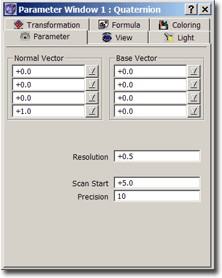### Quaternion ParametersHere you can define all parameters which describe the 3D space to calculate:
You can exactly specify the 3D space to use. Additionally you can specify, how this 3D space gets scanned by ChaosPro: Simply scanning the whole 3D space would be possible, but awfully slow. So I had to apply some logic in order to reduce calculation times.

Lets describe the parameters:

• Normal vector N and base vector V: These two 4D vectors describe a 3D space in normal form:
The 3D space consists of all 4D points P, which satisfy N·(P-V)=0

(where · means dot product: [a1,a2,a3,a4]·[b1,b2,b3,b4] = a1*b1+a2*b2+a3*b3+a4*b4 )

If you do not want to bother with mathematical meaning: Simply play around with the values: They affect the shape of the fractal.

The following parameters are somewhat difficult to explain: They are the result of a strange algorithm to scan the 3D space. The algorithm is “strange” because I had to speed up the calculation time.

• Resolution: As the name implies, this specifies some kind of resolution: To be more exact: It specifies the resolution in z-direction as a multiply of the fractal width (so on resizing the fractal you wont have to change this value: It will be adjusted according to the size of the fractal). For each pixel in the window ChaosPro calculates the ray which starts at the observer, goes through this pixel and ends at the transformation plane.
This ray gets examined using the specified resolution. In short terms: Increasing this value enhances the image quality and slows down calculation speed: If there are too many “holes” in the fractal, or if the fractal looks more like a collection of points rather than a solid object, then it is a good idea to increase the resolution.
• Start Scan:

This parameter helps to speed up the calculation:

Most of the time a Quaternion fractal is some smooth object. So if we want to examine a ray in order to find the border of a quaternion object and if we already know the distance of the neighbouring pixels, we can assume that the pixel in question nearly has the same distance.

So there is no need for ChaosPro to start searching at the beginning of the ray, i.e. at the observer. Instead, it can start at a distance near the neighbouring pixels. And that’s what this parameter is ment for:

Assume the neighbouring pixels are at distance d and Start Scan has been set to 10 (parameter is specified in percent, thus this means 10%).
Then ChaosPro starts scanning at d-d*Start Scan/100, or in other words, ChaosPro goes back 10% (=Start Scan) of the distance and starts scanning from there.

This greatly speeds up calculation times: Just watch the fractal calculation: The very first line of a quaternion, where *no* information about the fractal is available, needs much longer than all the succeeding lines, where this neighbouring information is available.

But the drawback is: Sudden big jumps in a quaternion object won’t be detected. So play around with this parameter, set it to 100 in order to “disable” the feature (this means: Go back 100%, i.e. to the observer, i.e. start from scratch…).

If you have a very strange object with many holes and cliffs and whatever, I would suggest to increase this parameter, although the calculation time then increases, too.

• Precision: The resolution basically should be at least about 20 times the fractal width.
If the resolution is set to 2, then the fractal object would seem to have “steps” in it, which disappear when the resolution is increased. If you want to see what I mean, choose Precision=1 and resolution=2, then increase the resolution. Do you see the steps? And do you see how they disappear when you increase the resolution?
Explanation: ChaosPro must determine the distance between a quaternion pixel and the observer. Lets say that ChaosPro scans a ray of length 4 at 200 points. That means, each 0.02 (=4/200) unit a pixel gets tested whether it belongs to the quaternion or not. But now imagine a pixel is 1.009283 units away!
ChaosPro would test whether the pixel 1 unit away belongs to the quaternion:
Result: no
Now ChaosPro continues and tests the pixel 1.02.
Result: yes

And due to this effect steps appear in the fractal when the resolution is too low.
So basically we need to increase the resolution by some magnitude, but we do not want to increase the calculation times.
So a trick gets applied:
After having found a pixel ChaosPro uses a second pass to determine the exact distance of that pixel: In short terms: When ChaosPro finds a pixel in step i, it goes back one step and scans the ray between step i-1 and i at Precision points.
Lets take the previous example:

ChaosPro scans a ray of length 4 at 200 points.
That means, each 0.02 (=4/200) unit a pixel gets tested whether it belongs to the quaternion or not.
Assume Precision has been set to 40.
Assume there is a pixel 1.009283 units away.

ChaosPro would test whether the pixel 1 unit away belongs to the quaternion:
Result: no
Now ChaosPro continues and tests the pixel 1.02 (next step).
Result: yes
Ok, so ChaosPro knows, the border of our quaternion is between 1 and 1.02. So ChaosPro takes this short line segment of length 0.02, divides it into 40 pieces (=Precision) and checks again.

Conclusion: Precision artificially increases the resolution. The scan resolution basically is Resolution * Precision * Width_of_fractal